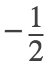# MATH 251: Calculus 3, SET8

## 13: Vectors Functions

### 13.3: Arc Length and Curvature

These problems are done with the CAS. See Hand Solutions for details.

#### 1. [868/4]

The arc length of a space curve is computed.
syms t
r = [cos(t) sin(t) log(cos(t))]
r =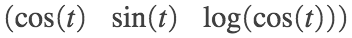Dr = diff(r,t)
Dr =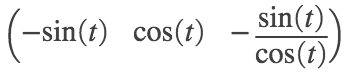f = simplify(sum(Dr.^2)) % or sec(t)^2
f =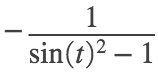g = sec(t) % sqrt(f) for 0 <= t <= pi/4
g =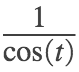L = int(g, t, 0, pi/4)
L =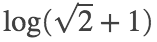L_appx = double(L) % in cm
L_appx = 0.8814

#### 2. [868/12]

Parameterize the curve of intersection then proceed as in #1.
syms t x y z
elliptical_cylinder = 4*x^2 + y^2 == 4
elliptical_cylinder =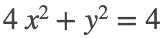plane = x + y + z == 2
plane =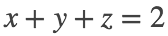r = [cos(t) 2*sin(t) 2-cos(t)-2*sin(t)]
r =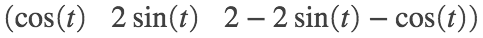Dr = diff(r,t)
Dr =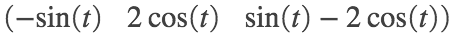g = sqrt(simplify(sum(Dr.^2)))
g =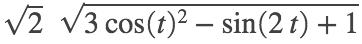L = int(g, t, 0, 2*pi)
L =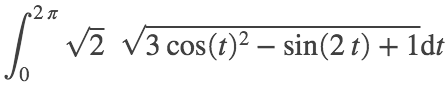L_appx = double(L) % in cm, to 4 decimal places
L_appx = 13.5191

#### 3. [868/18]

The unit tangent, unit normal, and curvature function for a space curve are computed.
syms t positive
r = [t^2 sin(t)-t*cos(t) cos(t)+t*sin(t)]
r =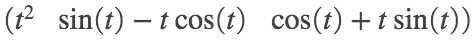Dr = diff(r,t)
Dr =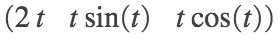mag_Dr = sqrt(simplify(sum(Dr.^2)))
mag_Dr =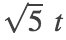T = Dr / mag_Dr % unit tangent vector
T =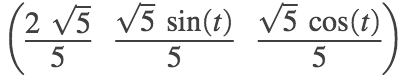DT = diff(T,t)
DT =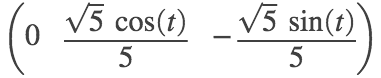mag_DT = sqrt(simplify(sum(DT.^2)))
mag_DT =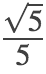N = DT / mag_DT % unit normal vector
N =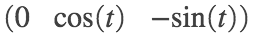kappa = mag_DT / mag_Dr % curvature
kappa =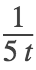#### 4. [868/24]

The curvature to a space curve at a point corresponding to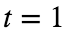is computed using Formula 10 on page 864.
syms t positive
r = [t^2 log(t) t*log(t)]
r =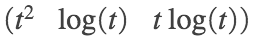Dr(t) = diff(r,t), D2r(t) = diff(Dr,t)
Dr(t) =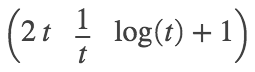D2r(t) =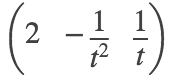v = Dr(1), a = D2r(1)
v =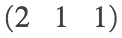a =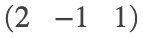kappa = simplify(norm(cross(v,a)) / norm(v)^3)
kappa =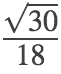kappa_appx = double(kappa)
kappa_appx = 0.3043

#### 5. [868/30]

We use Formula 11 on page 865 to analyze the curvature of a curve in the xy-plane.
syms x positive
f = log(x), Df = diff(f,x), D2f = diff(Df,x)
f =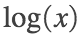Df =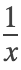D2f =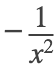k = simplify(abs(D2f) / (1 + Df^2)^(3/2))
k =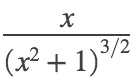Dk = simplify(diff(k,x))
Dk =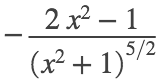xM = solve(Dk == 0, x) % Maximum curvature occurs at x = sqrt(2)/2 via FDT for extr vals.
xM =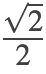L = limit(k, x, inf) % As x approaches infinity, the curvature approaches zero.
L = 0

#### 6. [869/36]

A parametric space curve and its curvature are graphed for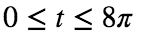on separate plots.
syms t positive
r = [t-sin(t) 1-cos(t) 4*cos(t/2)]
r =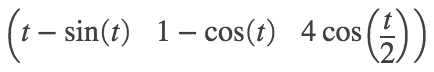Dr = diff(r,t);
mag_Dr = sqrt(simplify(sum(Dr.^2)));
T = Dr / mag_Dr; % unit tangent vector
DT = diff(T,t);
mag_DT = sqrt(simplify(sum(DT.^2)));
N = DT / mag_DT; % unit normal vector
kappa = mag_DT / mag_Dr % The curvature is obtained. (Intermediate results suppressed.)
kappa =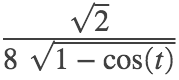%
figure
fplot3(t-sin(t), 1-cos(t), 4*cos(t/2), [0 8*pi], 'LineWidth', 2)
xlabel('x'); ylabel('y'); zlabel('z')
title('SET8, 869/36: space curve, viewed from above')
view(0,90) % View looking down the positive z-axis, straight onto the xy-plane.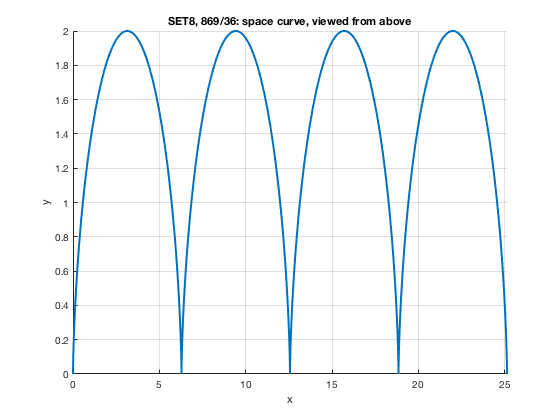figure
fplot(t, kappa, [0 8*pi], 'r', 'LineWidth', 2)
axis([0 8*pi 0 7]); grid on
xlabel('x'); ylabel('y')
title('SET8, 869/36: curvature')
ax = gca;
S = sym(ax.XLim(1):pi:ax.XLim(2));
ax.XTick = double(S);
ax.XTickLabel = arrayfun(@texlabel,S,'UniformOutput',false);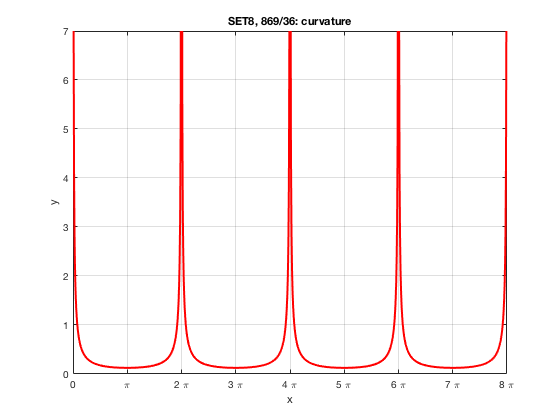% OBSERVATION:
% The curvature is minimal at odd multiples of pi
% and becomes infinite at even multiples of pi.

#### 7. [869/48]

Unit tangent, normal, and binormal vectors are computed at a point corresponding to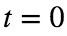on a space curve.
syms t
r = [cos(t), sin(t), log(cos(t))]
r =Dr = diff(r,t), Dr = [-sin(t) cos(t) -tan(t)]
Dr =Dr =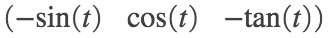mag_Dr = sec(t)
mag_Dr =T = simplify(Dr / mag_Dr) % unit tangent vector
T =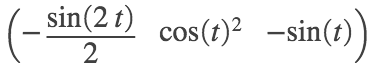DT = simplify(diff(T,t))
DT =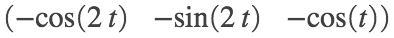mag_DT = sqrt(simplify(sum(DT.^2)))
mag_DT =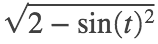N = DT / mag_DT % unit normal vector
N =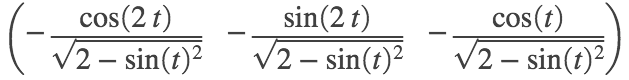% The unit binormal vector is B = T x N.
T0 = subs(T, t, 0), N0 = subs(N, t, 0), B0 = cross(T0,N0) % T, N, B for t = 0
T0 =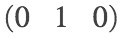N0 =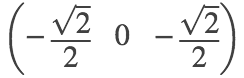B0 =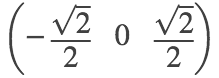#### 8. [869/53]

A point on a space curve is found whose normal plane is parallel to a given plane.
syms c t x y z
given_plane = 6*x + 6*y - 8*z == 1 % normal vector to given plane: [6 6 -8]
given_plane =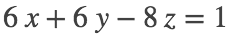r(t) = [t^3 3*t t^4], Dr(t) = diff(r(t),t)
r(t) =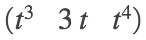Dr(t) =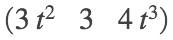% The tangent vector to the space curve is perpendicular to the normal plane.
% To be parallel to the given plane, it must be a multiple of [6 6 -8].
%
eqs = Dr(t) == c*[6 6 -8]
eqs =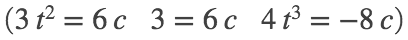s = solve(eqs, [c t])
s = struct with fields:
c: [1×1 sym] t: [1×1 sym]
sc = s.c, st = s.t
sc =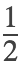st =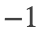P = r(-1), n = 1/2*[6 6 -8] % The desired point is (-1, -3, 1), at which norm vect is [3 3 -4].
P =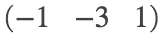n = 1×3
3 3 -4

#### 9. [870/64]

syms t real
syms a b positive
r = [a*cos(t) a*sin(t) b*t]
r =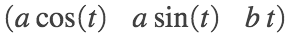Dr = diff(r,t), D2r = diff(Dr,t), D3r = diff(D2r,t)
Dr =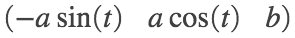D2r =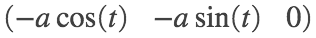D3r =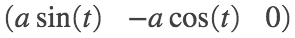mag_Dr = sqrt(simplify(sum(Dr.^2)));
T = Dr / mag_Dr % unit tangent vector
T =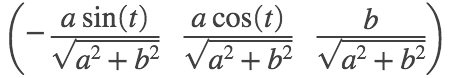DT = diff(T,t)
DT =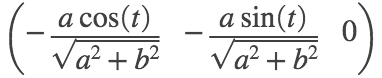mag_DT = sqrt(simplify(sum(DT.^2)));
N = DT / mag_DT % unit normal vector
N =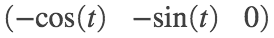kappa = mag_DT / mag_Dr % constant curvature
kappa =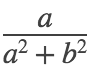c = cross(Dr,D2r)
c =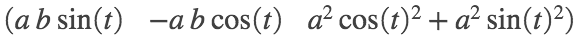tau = simplify(dot(c, D3r) / norm(c)^2) % constant torsion
tau =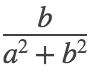#### 10. [870/66]

syms t real
r = [sinh(t) cosh(t) t] % = [0 1 0] when t = 0
r =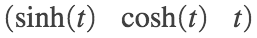Dr = diff(r,t), D2r = diff(Dr,t), D3r = diff(D2r,t)
Dr =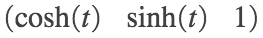D2r =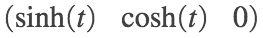D3r =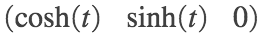%
kappa(t) = simplify(norm(cross(Dr,D2r)) / norm(Dr)^3)
kappa(t) =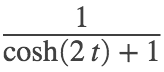kappa0 = kappa(0) % Curvature is 1/2 at (0,1,0).
kappa0 =%
c = cross(Dr,D2r)
c =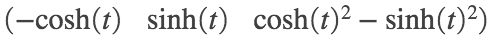tau(t) = simplify(dot(c, D3r) / norm(c)^2)
tau(t) =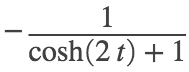tau0 = tau(0) % Torsion is -1/2 at (0,1,0).
tau0 =